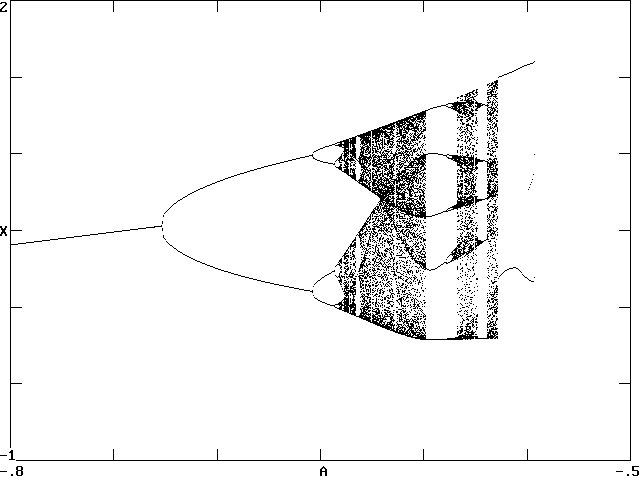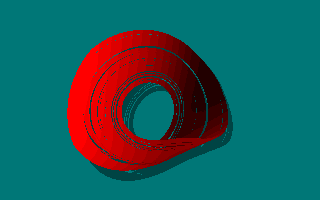# Simple Chaotic Flow with ABS Nonlinearity

J. C. Sprott
Department of Physics, University of Wisconsin, Madison, WI 53706, USA
September 22, 1998
Last revised March 11, 2004

Previous work has identified numerous examples of algebraically simple chaotic flows with quadratic nonlinearities.  Here we report the result of a search for simple chaotic flows in which the single nonlinearity has the form of the absolute value of one of the variables.  Such an example is to quadratic flows as the tent map is to the logistic map.

Following a suggestion by Stefan Linz, equations of the form d3x/dt3 = Ad2x/dt2 + Bdx/dt + C|x| + D were examined.  Many chaotic solutions were found for various choices of the parameters A, B, C, and D, and different initial conditions.  One simple example is d3x/dt3 = Ad2x/dt2 - dx/dt + |x| - 1 with initial conditions at x = dx/dt = d2x/dt2 = 0.

A bifurcation diagram for the above equation was produced using the BASIC source and (DOS) object code provided.  The parameter A was varied from -0.8 to -0.5, and the local maximum in x was plotted.  The resulting period-doubling route to chaos is shown below:A Hopf bifurcation occurs at A = -1 (off the left edge of the figure), where the stable fixed point at x = -1 bifurcates into a limit cycle with unit angular frequency.  For A = -0.6, variation of B and C reveal a similar period-doubling route to chaos.  Variation of D controls the size of the attractor and appears to produce chaotic solutions for all D < 0.  For A = -0.6 (just to the left of the period-5 window), the calculated Lyapunov exponents are (0.035, 0, -0.635), and the Kaplan-Yorke dimension is 2.055.  An animated view of the attractor with A = -0.6 is shown below:In the image above, the dx/dt axis is toward the top of the screen and the object rotates in the x-d2x/dt2 plane.  Lucas Finco has written a Java applet that allows you to vary A and rotate the attractor arbitrarily.  This system is ripe for further exploration.  The discontinuity in the flow is not evident in the image of the attractor because it occurs only in the fourth derivative (d4x/dt4).  The attractor is strikingly similar to other simple flows with quadratic nonlinearities.  Several other simple systems with the absolute value nonlinearity are also known.

An operational amplifier circuit that simulates the dynamics of the above system is given below:In this circuit, if all components except the variable resistor have unit magnitude (ohms, farads, and volts), the output should exactly solve the equation in real time.  Alternately, the resistors can be 1 kilo-ohm and the capacitors can be 1000 microfarads.  If the capacitors are decreased to 0.1 microfarad, the frequency should increase by a factor of 10,000 making the output in the audio range (1592 Hz at the first Hopf bifurcation).  This permits the bifurcations and chaos to be heard with a loudspeaker as the variable resistor (value 1/A) is adjusted from 1000 to 2000 ohms.  Germanium signal diodes should be used to reduce the forward voltage drop, although the circuit will work with silicon diodes at a slightly smaller value of A.

The circuit has been successfully simulated with SPICE using real operational amplifiers and diodes, and the only possible difficulty occurs if the right-most operational amplifier has a dc offset that produces a positive output in the absence of any input.  A real circuit using parts available for about \$10 from Radio Shack has also been constructed and tested.  Its output is available in WAV format and in RealAudio format as 1/A is increased.  The digitized signal is actually the integral of x so as to accentuate the low frequencies during the period doublings.  You should be able to hear the period-1, period-2, period-4, and period-8 bifurcations, chaos, the period-6 window, chaos again, the period-5 window with doubling to period-10, and chaos again.

Many other chaotic electrical circuits have been devised.  The virtue of this one is that all components are linear except for the diodes, and their nonlinearity is entirely in their I-V characteristic and not in some subtle and hard-to-model property such as junction capacitance.  For this reason, the circuit can be scaled to operate over a nearly arbitrary range of frequencies, and quantitative comparisons can be made with theory to the precision of the electrical components (a percent or better).  This raises the question of what is the simplest chaotic circuit that contains only linear operational amplifiers, resistors, capacitors, diodes, and batteries.  The circuit above has 18 components.  At least one other such circuit with 18 components has been devised.  Other circuits with as few as 11 components have recently been found and are under study.  A longer paper on chaotic circuits with more detail is also available.

The circuit is similar in spirit to Chua's circuit, which is also piecewise linear but uses an inductor and diodes or saturated operational amplifiers to provide a negative resistance.  The circuit here has a number of advantages over Chua's circuit:

1. It doesn't require inductors which are hard to model precisely because of their frequency dependent resistive loss.
2. It is less sensitive to the values and quality of the components.
3. It can be scaled to operate at essentially any frequency.
4. When the forward voltage drop of the diodes is taken into account, the circuit permits automated bifurcation plots using a swept voltage source in place of the battery.
5. It is a piecewise linear approximation to a quadratic rather than cubic nonlinearity.
6. Its representation in terms of a third order ODE is much simpler.
This work has been published as S. J. Linz and J. C. Sprott, Phys. Lett. A 259, 240-245 (1999).
The circuits are published in Phys. Lett. A and Am. J. Phys.
See also K. Kiers, D. Schmidt, and J. C. Sprott, Precision Measurements of a Simple Chaotic Circuit, Am. J. Phys. 72, 503-509 (2004).